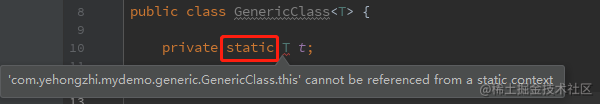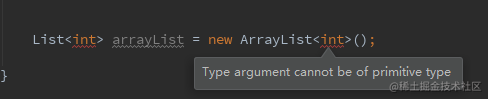# Java基础不简单，泛型很重要！# 前言

``````List<String> list = new ArrayList<>();
Map<String,Object> map = new HashMap<>();

# 什么是泛型

Java泛型是J2SE1.5中引入的一个新特性，其本质是参数化类型，也就是说所操作的数据类型被指定为一个参数（type parameter），这种参数类型可以用在类、接口和方法的创建中，分别称为泛型类、泛型接口、泛型方法。-- 百度百科

# 为什么使用泛型

``````public static void main(String[] args) {
List list = new ArrayList();
for(int i = 0; i < list.size(); i++){
String val = (String) list.get(i);
System.out.println("val:" + val);
}
}

• 类型安全问题。使用泛型，则会在编译期就能发现类型转换异常的错误。
• 消除类型强转。泛型可以消除源代码中的许多强转类型的操作，这样可以使代码更加可读，并减少出错的机会。

# 泛型的特性

``````List<String> stringArrayList = new ArrayList<String>();
List<Integer> integerArrayList = new ArrayList<Integer>();

Class classStringArrayList = stringArrayList.getClass();
Class classIntegerArrayList = integerArrayList.getClass();
System.out.println(classStringArrayList == classIntegerArrayList);

Java 泛型擦除是 Java 泛型中的一个重要特性，其目的是避免过多的创建类而造成的运行时的过度消耗。

# 泛型的使用方式

## 泛型类

``````public class 类名<泛型标识, 泛型标识, ...> {
private 泛型标识 变量名;
}

``````public class GenericClass<T> {
private T t;
}

``````public class GenericClass<T> {

private T t;

public GenericClass() {
}

public void setValue(T t) {//作为参数
this.t = t;
}

public T getValue() {//作为返回值
return t;
}
}

``````public static void main(String[] args) throws Exception {
//泛型传入了String类型
GenericClass<String> generic = new GenericClass<String>();
//这里就限制了setValue()方法只能传入String类型
generic.setValue("abc");
//限制了getValue()方法得到的也是String类型
String value = generic.getValue();
System.out.println(value);
}

## 泛型方法

``````修饰符 <T, E, ...> 返回值类型 方法名(形参列表){
}

``````//静态方法
public static <E> E getObject1(Class<E> clazz) throws Exception {
return clazz.newInstance();
}

//实例方法
public <E> E getObject2(Class<E> clazz) throws Exception {
return clazz.newInstance();
}

``````public static void main(String[] args) throws Exception {
GenericClass<String> generic = new GenericClass<>();
Object object1 = GenericClass.getObject1(Object.class);
System.out.println(object1);
Object object2 = generic.getObject2(Object.class);
System.out.println(object2);
}

``````public class GenericClass<T> {
private T t;

public GenericClass() {
}
//泛型类的方法
public void setValue(T t) {
this.t = t;
}
//泛型类的方法
public T getValue() {
return t;
}
//静态泛型方法，区别在于在返回值前需要加上尖括号<泛型标识>
public static <E> E getObject1(Class<E> clazz) throws Exception {
return clazz.newInstance();
}
//实例泛型方法，区别在于在返回值前需要加上尖括号<泛型标识>
public <E> E getObject2(Class<E> clazz) throws Exception {
return clazz.newInstance();
}
}

## 泛型接口

``````public 接口名称 <泛型标识, 泛型标识, ...>{
泛型标识 方法名();
}

``````public interface Generator<T> {
T next();
}

``````public class ObjectGenerator<T> implements Generator<T> {
@Override
public T next() {
return null;
}
}

``````public class IntegerGenerator implements Generator<Integer> {
@Override
public Integer next() {
Random random = new Random();
return random.nextInt(10);
}
}

## 泛型通配符

### 类型通配符上限

``````类/接口<? extends 实参类型>

``````public class ListUtils<T extends List> {
public void doing(T t) {
System.out.println("size: " + t.size());
for (Object o : t) {
System.out.println(o);
}
}
}

``````public static void main(String[] args) throws Exception {
ListUtils<List> utils = new ListUtils<>();
List<String> list = Arrays.asList("1", "2", "3");
utils.doing(list);
}

### 类型通配符下限

``````类/接口<? super 实参类型>

``````public class ListUtils {
//静态泛型方法，使用super关键字定义通配符下限
public static <E> void copy(Collection<? super E> parentList, Collection<E> childList) {
for (E e : childList) {
}
}
}

``````public static void main(String[] args) throws Exception {
Integer i1 = new Integer(10);
Integer i2 = new Integer(20);
List<Integer> integers = new ArrayList<>();
List<Number> numbers = new ArrayList<>();
//childList传入的是List<Integer>，所以parentList传入的只能是Integer本身或者其父类的List
ListUtils.copy(numbers, integers);
System.out.println(numbers);//[10, 20]
}

## 泛型使用注意点# 总结

• 提高了代码的可读性，这点毋庸置疑。
• 解决类型安全的问题，在编译期就能发现类型转换异常的错误。
• 可拓展性强，可以使用泛型通配符定义，不需要定义实际的数据类型。
• 提高了代码的重用性。可以重用数据处理算法，不需要为每一种类型都提供特定的代码。